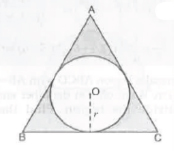# Areas related to Cirles-Important Questions

Class X Math
Hots for Areas Related to Circles
1.   In the figure, find teh perimeter of the shaded region where. ADC, AEB and BFC are semi-circles on diameters AC, AB and BC respectively.
2.   In the figure, arcs and drawn by taking vertices A, B and C of an equilateral triangle of side 10 cm to intersect the sides BC, CA and AB at their respective mid-points D, E and F. Find the area of teh shaded region. [use π = 3.14]
3.   In figure OABC is a quadrant of a circle of radius 7 cm. If OD = 4 cm, find the area of the shaded region.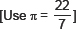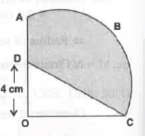4.   A chord of a circle of radius 20 cm subtends an angle of 90° at the centre. Find the area of the corresponding major segment of the circle.
5.   Area of a sector of a circle of radius 36 cm is 54π cm2. Find the length of the corresponding arc of the sector.
6.   In the figure, AB is a diameter of the circle, AC = 6 cm and BC = 8 cm. Find the area of the shaded region. [Use π = 3.14]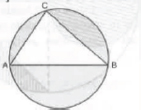7.   A calf is tied with a rope of length 6 m at the corner of a square grassy lawn of side 20 m. If the length of the rope is increased by 5.5 m, find the increase in area of the grassy lawn in which the calf can graze.
8.   In the figure, a circle is inscribed in an equilateral triangle ABC of side 12 cm. Find the radius of teh inscribed circle and the area of the shaded region. [Use π = 3.14 and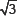= 1.73]